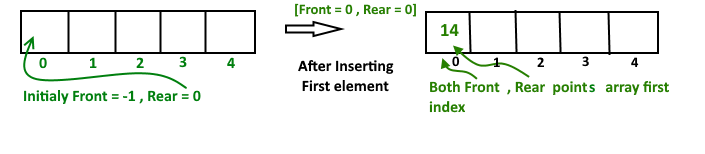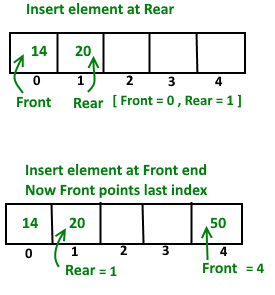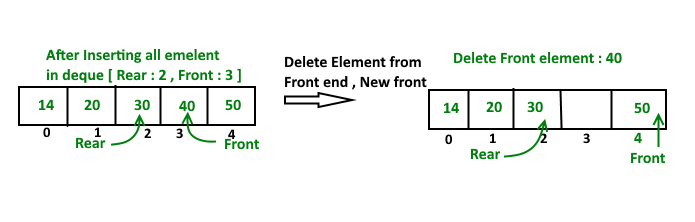# Implementation of Deque using circular array

• Difficulty Level : Easy
• Last Updated : 09 Mar, 2022

Deque or Double Ended Queue is a generalized version of Queue data structure that allows insert and delete at both ends.In previous post we had discussed introduction of deque. Now in this post we see how we implement deque Using circular array.
Operations on Deque:
Mainly the following four basic operations are performed on queue:
insertFront(): Adds an item at the front of Deque.
insertRear(): Adds an item at the rear of Deque.
deleteFront(): Deletes an item from front of Deque.
deleteRear(): Deletes an item from rear of Deque.
In addition to above operations, following operations are also supported
getFront(): Gets the front item from queue.
getRear(): Gets the last item from queue.
isEmpty(): Checks whether Deque is empty or not.
isFull(): Checks whether Deque is full or not.Circular array implementation deque
For implementing deque, we need to keep track of two indices, front and rear. We enqueue(push) an item at the rear or the front end of dequeue and dequeue(pop) an item from both rear and front end.
Working
1. Create an empty array ‘arr’ of size ‘n’
initialize front = -1 , rear = 0
Inserting First element in deque, at either front or rear will lead to the same result.After insert Front Points = 0 and Rear points = 0
Insert Elements at Rear end

```a). First we check deque if Full or Not
b). IF Rear == Size-1
then reinitialize Rear = 0 ;
Else increment Rear by '1'
and push current key into Arr[ rear ] = key
Front remain same.      ```

Insert Elements at Front end

```a). First we check deque if Full or Not
b). IF Front == 0 || initial position, move Front
to points last index of array
front = size - 1
Else decremented front by '1' and push
current key into Arr[ Front] = key
Rear remain same. ```Delete Element From Rear end

```a). first Check deque is Empty or Not
b).  If deque has only one element
front = -1 ; rear =-1 ;
Else IF Rear points to the first index of array
it's means we have to move rear to points
last index [ now first inserted element at
front end become rear end ]
rear = size-1 ;
Else || decrease rear by '1'
rear = rear-1;```

Delete Element From Front end

```a). first Check deque is Empty or Not
b).  If deque has only one element
front = -1 ; rear =-1 ;
Else IF front points to the last index of the array
it's means we have no more elements in array so
we move front to points first index of array
front = 0 ;
Else || increment Front by '1'
front = front+1;```Below is the implementation of above idea.

## C++

 `// C++ implementation of De-queue using circular``// array``#include``using` `namespace` `std;` `// Maximum size of array or Dequeue``#define MAX 100` `// A structure to represent a Deque``class` `Deque``{``    ``int`  `arr[MAX];``    ``int`  `front;``    ``int`  `rear;``    ``int`  `size;``public` `:``    ``Deque(``int` `size)``    ``{``        ``front = -1;``        ``rear = 0;``        ``this``->size = size;``    ``}` `    ``// Operations on Deque:``    ``void`  `insertfront(``int` `key);``    ``void`  `insertrear(``int` `key);``    ``void`  `deletefront();``    ``void`  `deleterear();``    ``bool`  `isFull();``    ``bool`  `isEmpty();``    ``int`  `getFront();``    ``int`  `getRear();``};` `// Checks whether Deque is full or not.``bool` `Deque::isFull()``{``    ``return` `((front == 0 && rear == size-1)||``            ``front == rear+1);``}` `// Checks whether Deque is empty or not.``bool` `Deque::isEmpty ()``{``    ``return` `(front == -1);``}` `// Inserts an element at front``void` `Deque::insertfront(``int` `key)``{``    ``// check whether Deque if  full or not``    ``if` `(isFull())``    ``{``        ``cout << ``"Overflow\n"` `<< endl;``        ``return``;``    ``}` `    ``// If queue is initially empty``    ``if` `(front == -1)``    ``{``        ``front = 0;``        ``rear = 0;``    ``}` `    ``// front is at first position of queue``    ``else` `if` `(front == 0)``        ``front = size - 1 ;` `    ``else` `// decrement front end by '1'``        ``front = front-1;` `    ``// insert current element into Deque``    ``arr[front] = key ;``}` `// function to inset element at rear end``// of Deque.``void` `Deque ::insertrear(``int` `key)``{``    ``if` `(isFull())``    ``{``        ``cout << ``" Overflow\n "` `<< endl;``        ``return``;``    ``}` `    ``// If queue is initially empty``    ``if` `(front == -1)``    ``{``        ``front = 0;``        ``rear = 0;``    ``}` `    ``// rear is at last position of queue``    ``else` `if` `(rear == size-1)``        ``rear = 0;` `    ``// increment rear end by '1'``    ``else``        ``rear = rear+1;` `    ``// insert current element into Deque``    ``arr[rear] = key ;``}` `// Deletes element at front end of Deque``void` `Deque ::deletefront()``{``    ``// check whether Deque is empty or not``    ``if` `(isEmpty())``    ``{``        ``cout << ``"Queue Underflow\n"` `<< endl;``        ``return` `;``    ``}` `    ``// Deque has only one element``    ``if` `(front == rear)``    ``{``        ``front = -1;``        ``rear = -1;``    ``}``    ``else``        ``// back to initial position``        ``if` `(front == size -1)``            ``front = 0;` `        ``else` `// increment front by '1' to remove current``            ``// front value from Deque``            ``front = front+1;``}` `// Delete element at rear end of Deque``void` `Deque::deleterear()``{``    ``if` `(isEmpty())``    ``{``        ``cout << ``" Underflow\n"` `<< endl ;``        ``return` `;``    ``}` `    ``// Deque has only one element``    ``if` `(front == rear)``    ``{``        ``front = -1;``        ``rear = -1;``    ``}``    ``else` `if` `(rear == 0)``        ``rear = size-1;``    ``else``        ``rear = rear-1;``}` `// Returns front element of Deque``int` `Deque::getFront()``{``    ``// check whether Deque is empty or not``    ``if` `(isEmpty())``    ``{``        ``cout << ``" Underflow\n"` `<< endl;``        ``return` `-1 ;``    ``}``    ``return` `arr[front];``}` `// function return rear element of Deque``int` `Deque::getRear()``{``    ``// check whether Deque is empty or not``    ``if``(isEmpty() || rear < 0)``    ``{``        ``cout << ``" Underflow\n"` `<< endl;``        ``return` `-1 ;``    ``}``    ``return` `arr[rear];``}` `// Driver program to test above function``int` `main()``{``    ``Deque dq(5);``    ``cout << ``"Insert element at rear end  : 5 \n"``;``    ``dq.insertrear(5);` `    ``cout << ``"insert element at rear end : 10 \n"``;``    ``dq.insertrear(10);` `    ``cout << ``"get rear element "` `<< ``" "``         ``<< dq.getRear() << endl;` `    ``dq.deleterear();``    ``cout << ``"After delete rear element new rear"``         ``<< ``" become "` `<< dq.getRear() << endl;` `    ``cout << ``"inserting element at front end \n"``;``    ``dq.insertfront(15);` `    ``cout << ``"get front element "` `<< ``" "``         ``<< dq.getFront() << endl;` `    ``dq.deletefront();` `    ``cout << ``"After delete front element new "``       ``<< ``"front become "` `<< dq.getFront() << endl;``    ``return` `0;``}`

## Java

 `// Java implementation of De-queue using circular``// array`` ` `// A structure to represent a Deque``class` `Deque``{``    ``static` `final` `int` `MAX = ``100``;``    ``int`  `arr[];``    ``int`  `front;``    ``int`  `rear;``    ``int`  `size;``    ` `    ``public` `Deque(``int` `size)``    ``{``        ``arr = ``new` `int``[MAX];``        ``front = -``1``;``        ``rear = ``0``;``        ``this``.size = size;``    ``}`` ` `    ``/*// Operations on Deque:``    ``void  insertfront(int key);``    ``void  insertrear(int key);``    ``void  deletefront();``    ``void  deleterear();``    ``bool  isFull();``    ``bool  isEmpty();``    ``int  getFront();``    ``int  getRear();*/`` ` `    ``// Checks whether Deque is full or not.``    ``boolean` `isFull()``    ``{``        ``return` `((front == ``0` `&& rear == size-``1``)||``            ``front == rear+``1``);``    ``}`` ` `    ``// Checks whether Deque is empty or not.``    ``boolean` `isEmpty ()``    ``{``        ``return` `(front == -``1``);``    ``}`` ` `    ``// Inserts an element at front``    ``void` `insertfront(``int` `key)``    ``{``        ``// check whether Deque if  full or not``        ``if` `(isFull())``        ``{``            ``System.out.println(``"Overflow"``);``            ``return``;``        ``}`` ` `        ``// If queue is initially empty``        ``if` `(front == -``1``)``        ``{``            ``front = ``0``;``            ``rear = ``0``;``        ``}``        ` `        ``// front is at first position of queue``        ``else` `if` `(front == ``0``)``            ``front = size - ``1` `;`` ` `        ``else` `// decrement front end by '1'``            ``front = front-``1``;`` ` `        ``// insert current element into Deque``        ``arr[front] = key ;``    ``}`` ` `    ``// function to inset element at rear end``    ``// of Deque.``    ``void` `insertrear(``int` `key)``    ``{``        ``if` `(isFull())``        ``{``            ``System.out.println(``" Overflow "``);``            ``return``;``        ``}`` ` `        ``// If queue is initially empty``        ``if` `(front == -``1``)``        ``{``            ``front = ``0``;``            ``rear = ``0``;``        ``}`` ` `        ``// rear is at last position of queue``        ``else` `if` `(rear == size-``1``)``            ``rear = ``0``;`` ` `        ``// increment rear end by '1'``        ``else``            ``rear = rear+``1``;``        ` `        ``// insert current element into Deque``        ``arr[rear] = key ;``    ``}`` ` `    ``// Deletes element at front end of Deque``    ``void` `deletefront()``    ``{``        ``// check whether Deque is empty or not``        ``if` `(isEmpty())``        ``{``            ``System.out.println(``"Queue Underflow\n"``);``            ``return` `;``        ``}`` ` `        ``// Deque has only one element``        ``if` `(front == rear)``        ``{``            ``front = -``1``;``            ``rear = -``1``;``        ``}``        ``else``            ``// back to initial position``            ``if` `(front == size -``1``)``                ``front = ``0``;`` ` `            ``else` `// increment front by '1' to remove current``                ``// front value from Deque``                ``front = front+``1``;``    ``}`` ` `    ``// Delete element at rear end of Deque``    ``void` `deleterear()``    ``{``        ``if` `(isEmpty())``        ``{``            ``System.out.println(``" Underflow"``);``            ``return` `;``        ``}`` ` `        ``// Deque has only one element``        ``if` `(front == rear)``        ``{``            ``front = -``1``;``            ``rear = -``1``;``        ``}``        ``else` `if` `(rear == ``0``)``            ``rear = size-``1``;``        ``else``            ``rear = rear-``1``;``    ``}`` ` `    ``// Returns front element of Deque``    ``int` `getFront()``    ``{``        ``// check whether Deque is empty or not``        ``if` `(isEmpty())``        ``{``            ``System.out.println(``" Underflow"``);``            ``return` `-``1` `;``        ``}``        ``return` `arr[front];``    ``}`` ` `    ``// function return rear element of Deque``    ``int` `getRear()``    ``{``        ``// check whether Deque is empty or not``        ``if``(isEmpty() || rear < ``0``)``        ``{``            ``System.out.println(``" Underflow\n"``);``            ``return` `-``1` `;``        ``}``        ``return` `arr[rear];``    ``}`` ` `    ``// Driver program to test above function``    ``public` `static` `void` `main(String[] args)``    ``{``        ` `         ``Deque dq = ``new` `Deque(``5``);``         ` `         ``System.out.println(``"Insert element at rear end  : 5 "``);``         ``dq.insertrear(``5``);``         ` `         ``System.out.println(``"insert element at rear end : 10 "``);``         ``dq.insertrear(``10``);``         ` `         ``System.out.println(``"get rear element : "``+ dq.getRear());``         ` `         ``dq.deleterear();``         ``System.out.println(``"After delete rear element new rear become : "` `+``                                ``dq.getRear());``         ` `         ``System.out.println(``"inserting element at front end"``);``         ``dq.insertfront(``15``);``         ` `         ``System.out.println(``"get front element: "` `+dq.getFront());``         ` `         ``dq.deletefront();``         ` `         ``System.out.println(``"After delete front element new front become : "` `+``                                    ``+  dq.getFront());``        ` `    ``}``}`

## Python3

 `# Python implementation of De-queue using circular``# array``  ` `# A structure to represent a Deque``MAX` `=` `100``;` `class` `Deque:``    ``def` `__init__(``self``, size):``        ``self``.arr ``=` `[``0``] ``*` `MAX``        ``self``.front ``=` `-``1``;``        ``self``.rear ``=` `0``;``        ``self``.size ``=` `size;``    ` `    ` `    ``''' Operations on Deque:``    ``void  insertfront(int key);``    ``void  insertrear(int key);``    ``void  deletefront();``    ``void  deleterear();``    ``bool  isFull();``    ``bool  isEmpty();``    ``int  getFront();``    ``int  getRear(); '''``  ` `    ``# Checks whether Deque is full or not.``    ``def` `isFull(``self``):``        ``return` `((``self``.front ``=``=` `0` `and` `self``.rear ``=``=` `self``.size``-``1``) ``or` `self``.front ``=``=` `self``.rear ``+` `1``)``    ` `    ` `    ``# Checks whether Deque is empty or not.``    ``def` `isEmpty (``self``):``        ``return` `(``self``.front ``=``=` `-``1``);``    ` `    ``# Inserts an element at front``    ``def` `insertfront(``self``, key):` `        ``# check whether Deque if  full or not``        ``if` `(``self``.isFull()):``            ``print``(``"Overflow"``);``            ``return``;``        ` `  ` `        ``# If queue is initially empty``        ``if` `(``self``.front ``=``=` `-``1``):``            ``self``.front ``=` `0``;``            ``self``.rear ``=` `0``;``        ` `         ` `        ``# front is at first position of queue``        ``elif` `(``self``.front ``=``=` `0``):``            ``self``.front ``=` `self``.size ``-` `1` `;``  ` `        ``else``: ``# decrement front end by '1'``            ``self``.front ``=` `self``.front``-``1``;``  ` `        ``# insert current element into Deque``        ``self``.arr[``self``.front] ``=` `key ;``    ` `    ` `    ``# function to inset element at rear end``    ``# of Deque.``    ``def` `insertrear(``self``, key):``        ``if` `(``self``.isFull()):``            ``print``(``" Overflow"``);``            ``return``;``        ` `  ` `        ``# If queue is initially empty``        ``if` `(``self``.front ``=``=` `-``1``):``            ``self``.front ``=` `0``;``            ``self``.rear ``=` `0``;``        ` `  ` `        ``# rear is at last position of queue``        ``elif` `(``self``.rear ``=``=` `self``.size``-``1``):``            ``self``.rear ``=` `0``;``  ` `        ``# increment rear end by '1'``        ``else``:``            ``self``.rear ``=` `self``.rear``+``1``;``         ` `        ``# insert current element into Deque``        ``self``.arr[``self``.rear] ``=` `key ;``    ` `    ` `    ``# Deletes element at front end of Deque``    ``def` `deletefront(``self``):``        ``# check whether Deque is empty or not``        ``if` `(``self``.isEmpty()):``            ``print``(``"Queue Underflow"``);``            ``return` `;``        ` `  ` `        ``# Deque has only one element``        ``if` `(``self``.front ``=``=` `self``.rear):``            ``self``.front ``=` `-``1``;``            ``self``.rear ``=` `-``1``;``        ` `        ``else``:``            ``# back to initial position``            ``if` `(``self``.front ``=``=` `self``.size ``-``1``):``                ``self``.front ``=` `0``;``  ` `            ``else``: ``# increment front by '1' to remove current``                ``# front value from Deque``                ``self``.front ``=` `self``.front``+``1``;``    ` `    ` `    ``# Delete element at rear end of Deque``    ``def` `deleterear(``self``):``        ``if` `(``self``.isEmpty()):``            ``print``(``" Underflow"``);``            ``return` `;``        ` `  ` `        ``# Deque has only one element``        ``if` `(``self``.front ``=``=` `self``.rear):``            ``self``.front ``=` `-``1``;``            ``self``.rear ``=` `-``1``;``        ` `        ``elif` `(``self``.rear ``=``=` `0``):``            ``self``.rear ``=` `self``.size``-``1``;``        ``else``:``            ``self``.rear ``=` `self``.rear``-``1``;``    ` `    ` `    ``# Returns front element of Deque``    ``def` `getFront(``self``):``        ``# check whether Deque is empty or not``        ``if` `(``self``.isEmpty()):``            ``print``(``" Underflow"``);``            ``return` `-``1` `;``        ` `        ``return` `self``.arr[``self``.front];``    ` `    ` `    ``# function return rear element of Deque``    ``def` `getRear(``self``):``        ``# check whether Deque is empty or not``        ``if``(``self``.isEmpty() ``or` `self``.rear < ``0``):``            ``print``(``" Underflow"``);``            ``return` `-``1` `;``        ` `        ``return` `self``.arr[``self``.rear];``    ` `    `   `# Driver program to test above function``dq ``=` `Deque(``5``);``          ` `print``(``"Insert element at rear end  : 5 "``);``dq.insertrear(``5``);` `print``(``"insert element at rear end : 10 "``);``dq.insertrear(``10``);` `print``(f``"get rear element : {dq.getRear()}"``);` `dq.deleterear();``print``(f``"After delete rear element new rear become : {dq.getRear()}"``);` `print``(``"inserting element at front end"``);``dq.insertfront(``15``);` `print``(f``"get front element: {dq.getFront()}"``);` `dq.deletefront();` `print``(f``"After delete front element new front become : {dq.getFront()}"``);` `# This code is contributed by _saurabh_jaiswal`

## C#

 `// C# implementation of De-queue using circular``// array``using` `System;` `// A structure to represent a Deque``public` `class` `Deque``{``    ``static` `readonly` `int` `MAX = 100;``    ``int`  `[]arr;``    ``int`  `front;``    ``int`  `rear;``    ``int`  `size;``    ` `    ``public` `Deque(``int` `size)``    ``{``        ``arr = ``new` `int``[MAX];``        ``front = -1;``        ``rear = 0;``        ``this``.size = size;``    ``}`` ` `    ``/*// Operations on Deque:``    ``void  insertfront(int key);``    ``void  insertrear(int key);``    ``void  deletefront();``    ``void  deleterear();``    ``bool  isFull();``    ``bool .Count!=0;``    ``int  getFront();``    ``int  getRear();*/`` ` `    ``// Checks whether Deque is full or not.``    ``bool` `isFull()``    ``{``        ``return` `((front == 0 && rear == size - 1)||``            ``front == rear + 1);``    ``}`` ` `    ``// Checks whether Deque is empty or not.``    ``bool` `isEmpty ()``    ``{``        ``return` `(front == -1);``    ``}`` ` `    ``// Inserts an element at front``    ``void` `insertfront(``int` `key)``    ``{``      ` `        ``// check whether Deque if  full or not``        ``if` `(isFull())``        ``{``            ``Console.WriteLine(``"Overflow"``);``            ``return``;``        ``}`` ` `        ``// If queue is initially empty``        ``if` `(front == -1)``        ``{``            ``front = 0;``            ``rear = 0;``        ``}``        ` `        ``// front is at first position of queue``        ``else` `if` `(front == 0)``            ``front = size - 1 ;`` ` `        ``else` `// decrement front end by '1'``            ``front = front - 1;`` ` `        ``// insert current element into Deque``        ``arr[front] = key ;``    ``}`` ` `    ``// function to inset element at rear end``    ``// of Deque.``    ``void` `insertrear(``int` `key)``    ``{``        ``if` `(isFull())``        ``{``            ``Console.WriteLine(``" Overflow "``);``            ``return``;``        ``}`` ` `        ``// If queue is initially empty``        ``if` `(front == -1)``        ``{``            ``front = 0;``            ``rear = 0;``        ``}`` ` `        ``// rear is at last position of queue``        ``else` `if` `(rear == size - 1)``            ``rear = 0;`` ` `        ``// increment rear end by '1'``        ``else``            ``rear = rear+1;``        ` `        ``// insert current element into Deque``        ``arr[rear] = key ;``    ``}`` ` `    ``// Deletes element at front end of Deque``    ``void` `deletefront()``    ``{``      ` `        ``// check whether Deque is empty or not``        ``if` `(isEmpty())``        ``{``            ``Console.WriteLine(``"Queue Underflow\n"``);``            ``return` `;``        ``}`` ` `        ``// Deque has only one element``        ``if` `(front == rear)``        ``{``            ``front = -1;``            ``rear = -1;``        ``}``        ``else``            ``// back to initial position``            ``if` `(front == size - 1)``                ``front = 0;`` ` `            ``else` `// increment front by '1' to remove current``                ``// front value from Deque``                ``front = front + 1;``    ``}`` ` `    ``// Delete element at rear end of Deque``    ``void` `deleterear()``    ``{``        ``if` `(isEmpty())``        ``{``            ``Console.WriteLine(``" Underflow"``);``            ``return` `;``        ``}`` ` `        ``// Deque has only one element``        ``if` `(front == rear)``        ``{``            ``front = -1;``            ``rear = -1;``        ``}``        ``else` `if` `(rear == 0)``            ``rear = size - 1;``        ``else``            ``rear = rear - 1;``    ``}`` ` `    ``// Returns front element of Deque``    ``int` `getFront()``    ``{``        ``// check whether Deque is empty or not``       ``if` `(isEmpty())``        ``{``            ``Console.WriteLine(``" Underflow"``);``            ``return` `-1 ;``        ``}``        ``return` `arr[front];``    ``}`` ` `    ``// function return rear element of Deque``    ``int` `getRear()``    ``{``      ` `        ``// check whether Deque is empty or not``       ``if` `(isEmpty() || rear < 0)``        ``{``            ``Console.WriteLine(``" Underflow\n"``);``            ``return` `-1 ;``        ``}``        ``return` `arr[rear];``    ``}`` ` `    ``// Driver code``    ``public` `static` `void` `Main(String[] args)``    ``{``        ` `         ``Deque dq = ``new` `Deque(5);      ``         ``Console.WriteLine(``"Insert element at rear end  : 5 "``);``         ``dq.insertrear(5);       ``         ``Console.WriteLine(``"insert element at rear end : 10 "``);``         ``dq.insertrear(10);      ``         ``Console.WriteLine(``"get rear element : "``+ dq.getRear());      ``         ``dq.deleterear();``         ``Console.WriteLine(``"After delete rear element new rear become : "` `+``                                ``dq.getRear());       ``         ``Console.WriteLine(``"inserting element at front end"``);``         ``dq.insertfront(15);    ``         ``Console.WriteLine(``"get front element: "` `+dq.getFront());     ``         ``dq.deletefront();    ``         ``Console.WriteLine(``"After delete front element new front become : "` `+``                                    ``+  dq.getFront());``    ``}``}` `// This code is contributed by aashish1995`

## Javascript

 ``

Output:

```insert element at rear end  : 5
insert element at rear end : 10
get rear element : 10
After delete rear element new rear become : 5
inserting element at front end
get front element : 15
After delete front element new front become : 5```

Time Complexity: Time complexity of all operations like insertfront(), insertlast(), deletefront(), deletelast()is O(1).
In next post we will discuss deque implementation using Doubly linked list.
This article is contributed by Nishant Singh. If you like GeeksforGeeks and would like to contribute, you can also write an article using write.geeksforgeeks.org or mail your article to review-team@geeksforgeeks.org. See your article appearing on the GeeksforGeeks main page and help other Geeks.